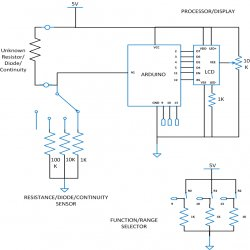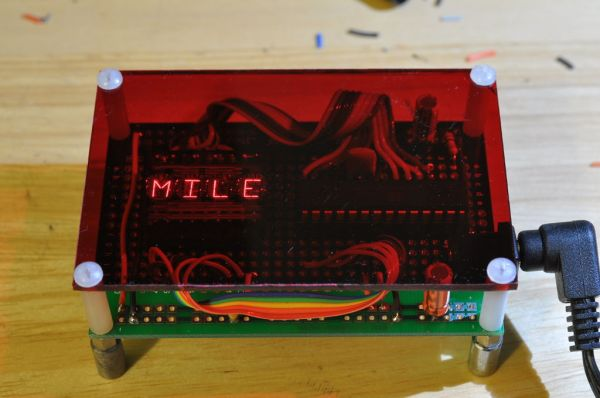# DIY: Arduino Based Ohmmeter

This is a project based on Arduino board which can measure the unknown resistance values and perform diode test and continuity testing. When we connect the unknown resistor on the breadboard circuit, the 16×2 LCD displays the resistor value and when we connect a diode the LCD displays the type of diode if it is a good one. When we take the probes out from the breadboard and connect it across a continuous path, the meter indicates the continuity by blinking an LED.The project uses an Arduino pro mini board whose ADC feature is used along with the concept of Voltage Divider circuit to develop this Ohmmeter.

Architecture of the project

The entire project can be divided into three basic blocks;

1)      Resistance/Diode/Continuity Sensor Unit

2)      Processor Unit

3)      Display Unit

The Sensor Unit takes three inputs, Unknown resistor, Diode and a Continuous path to be detected. When the unknown resistor is connected as input, the output voltage varies between 0 and 5V proportional to the value of resistance.  When the unknown diode is connected as input, the output voltage will be 4.3V for Silicon diode and 4.7V for Germanium diode. When a continuous path is connected as input, the output voltage will be 5V.The Processor Unit takes input voltage in the range of 0 to 5V. This unit calculates the resistance value, detects the diode type and continuous path from the input voltage value. The unit then sendsa 4bit data to the Display Unit which includes the resistance value or diode type. The Processor Unit also glows an LED if it detect a continuous path.

The Display Unit takes the 4bit data from the Processor Unit and produces a 16*2 display for the resistance value, diode type.

For more detail: DIY: Arduino Based Ohmmeter

#### This Post / Project can also be found using search terms:

• led arduino projects
• diy solder station arduino nano
• diy ohmmeter
• DIY: Arduino Based ohmmeter